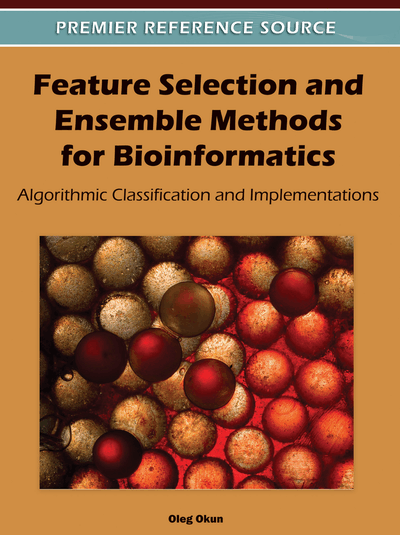# Nearest Neighbor

DOI: 10.4018/978-1-60960-557-5.ch005
Available
\$37.50
No Current Special Offers

Top

## Nearest Neighbor Classification

As follows from its name, a nearest neighbor (NN) classifier assigns a class label according to proximity or distance. The basic principle of this classifier is probably one of the easiest to comprehend: the closest neighbor of a given test instance (feature vector of gene expression levels) in the training set determines class membership of this test instance. So, basically, all that someone needs is distance computation (according to chosen distance metric) to a given test instance from every training instance. The shortest distance points to the neighbor whose class is assigned to the test instance. As one can see, the training phase is absent, which makes things even easier. Since only one neighbor decides on class membership, such a classifier is often called a 1-NN.

A natural extension of this idea is k-nearest neighbor (k-NN) classification, where k shortest distances are found and the label of a majority class is assigned to a test instance. In other words, the most common class among k nearest neighbors of an instance determines its class. The value of k is of importance as it influences on the classification results. Usually, it is chosen to be 1, 3, 5…, i.e. an odd number in order to avoid ties and decision uncertainties related to them1.

Both 1-NN and k-NN classifications are graphically depicted in Figure 1, where a 5-point star denotes a test instance to be classified and black and empty circles correspond to the training instances of two different classes. In Figure 1 (left) the class of a test point is ‘black circles’ as one of these circles lies at the shortest distance from the test point, whereas in Figure 1 (right) the class of the test point changes to ‘empty circles’ in 5-NN classification.

When dealing with high dimensional data such as gene expressions, a word of caution should be taken into account: in high dimensional spaces, the data points “live” close to the edges of the data set (see (Hastie, Tibshirani, & Friedman, 2001), pp. 22-23), which means that as a data dimensionality (the number of features) grows, the distance to the nearest data point approaches to the distance to the farthest data point (Beyer, Goldstein, Ramakrishnan, & Shaft, 1999), (Steinbach & Tan, 2009). This fact does not make NN classifiers meaningless but simply implies that dimensionality reduction has to take place prior to NN classification as irrelevant or noisy features can severely affect the classification accuracy of NN algorithms. The dimensionality reduction methods that are described in this book are considered to be useful for reducing the harmful effect of noise and irrelevant/redundant features.

Many NN algorithms have been proposed in the literature. For the state-of-the-art overview in early days, the book (Dasarathy, 1990) provides a good introduction. Although microarray data sets are rather small in the number of samples, the recent book (Shakhnarovich, Darrell, & Indyk, 2006) can serve as a reference to how fast NN search can be done for large and huge data sets.

The 1-NN has a strong theoretical property: as the data set size increases2, the 1-NN algorithm is guaranteed to yield the upper bounded error rate no more than twice the achievable optimum (Cover & Hart, 1967). This optimum is called the Bayes error rate (Duda, Hart, & Stork, 2000) and it is the minimum achievable error rate given the distribution of the data3.

In other words, ifandare the 1-NN error rate and the Bayes error rate, respectively, then the following inequality holds as(N is the training set size):. For the proof of this statement and a more formal definition of the Bayes error, see, for example, (Duda, Hart, & Stork, 2000) or http://cgm.cs.mcgill.ca/~soss/cs644/projects/simard/nn_prob_err.html.

## Complete Chapter List

Search this Book:
Reset